• 熵真是一个神奇的东西，据说之所以把它命名为熵就是因为它难以理解 但是它确实是一个很有用的西东，光机器学习里面，就经常见到它的身影，决策树要用到...交叉熵误差： 为实际的分类结果，为预测的结果，（并且...
熵真是一个神奇的东西，据说之所以把它命名为熵就是因为它难以理解
但是它确实是一个很有用的西东，光机器学习里面，就经常见到它的身影，决策树要用到它，神经网络和logistic回归也用到了它。
先说熵的定义：
熵定义为信息的期望，某个待分类事物可以划分为多个类别，其中类别的信息为(为的概率)：

熵为所有类别的信息期望值：
交叉熵误差：

为实际的分类结果， 为预测的结果，（ 并且   ）

这个函数被作为神经网络和logistic 回归的损失函数，因为它有三个很好的性质：
1. 它可以真实的反应出真实分类结果和预测结果的误差
假设, 即真实的分类结果是 , 则 交叉熵误差可以简化为：

函数图像如下：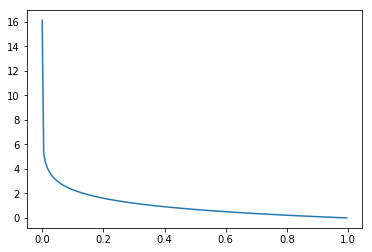可以看到， 越接近 ，即预测结果和真实分类结果越接近，误差越接近， 即误差越小

2. 交叉熵误差函数和softmax（神经网络用到的输出函数）和sigmoid函数（logistic回归用到的函数）的复合函数是凸函数，即存在全局最优解
凸函数的充要条件是：如果二阶导数存在，二阶到大于 ，现在以softmax函数为例证明：
softmax函数定义：

输入为  输出为
交叉熵误差函数：

两个复合函数对求一阶偏导：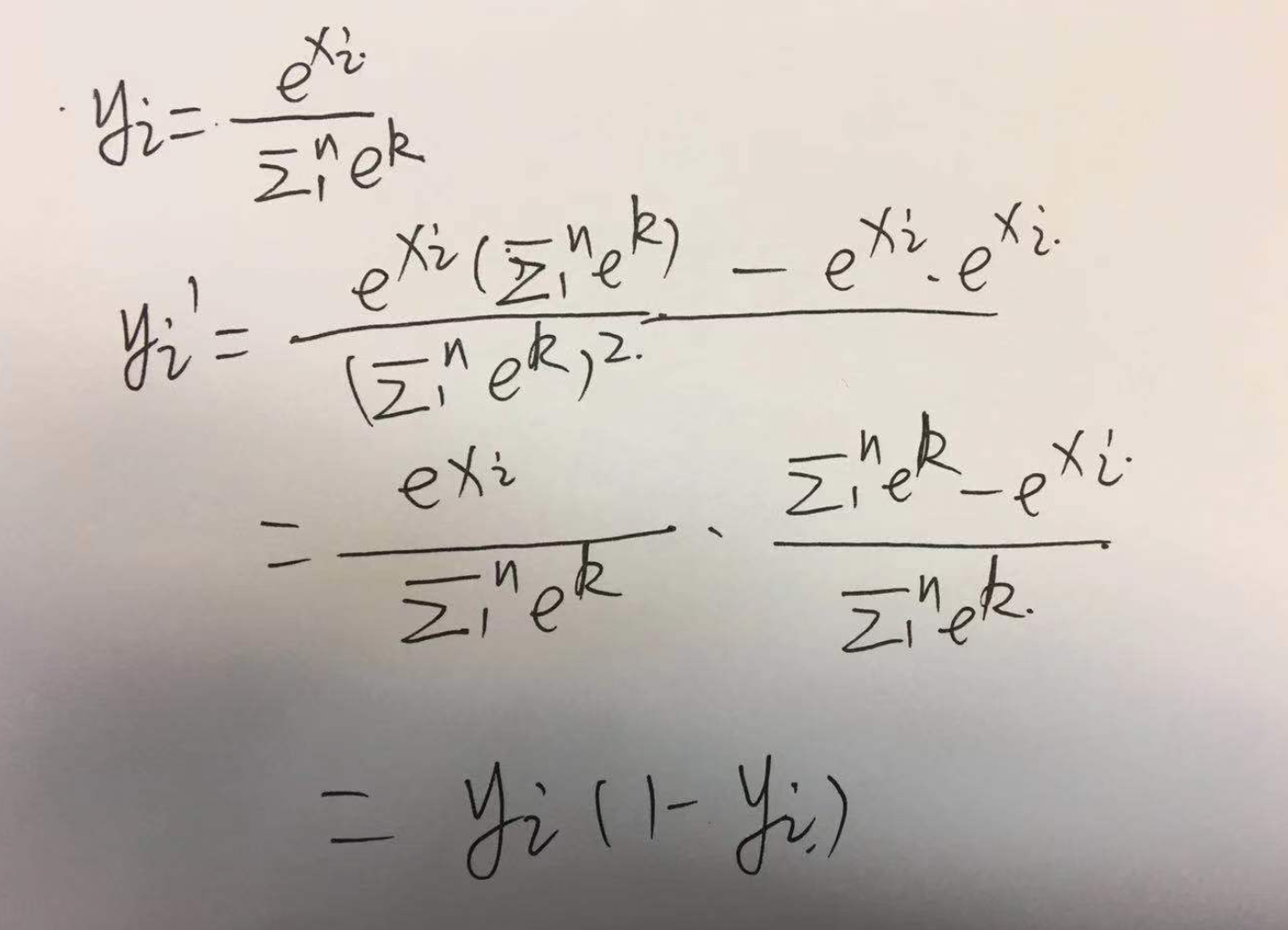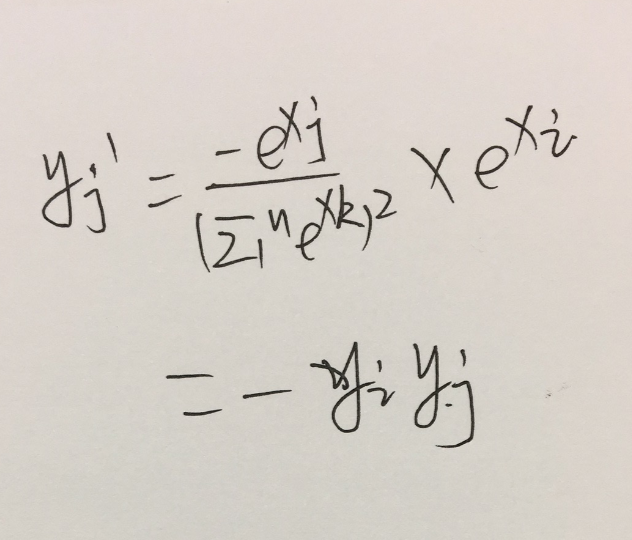所以：

二阶导数：

由于 上面的结果恒大于0， 二阶导数恒大于0 所以它是凸函数

3. 在用梯度下降发求解最优解时，需要用到一阶导数，从上面可以看到一阶导数：

很简洁漂亮，可以简化整个求解过程

数学真的很神奇！

reference:
机器学习实战：peter harrington（美）
深度学习入门：基于python的理论与实现  斋藤康毅（日）
展开全文机器学习 神经网络 信息熵
• mini-batch版交叉熵误差 深度学习入门：基于Python的理论与实现 # 可以同时处理单个数据和批量数据 def cross_entropy_error(y, t): '''判断维数，将单个数据和批量数据处理成同样的形式， 方便后面shape取出...
深度学习入门：基于Python的理论与实现
# 可以同时处理单个数据和批量数据
def cross_entropy_error(y, t):
'''判断维数，将单个数据和批量数据处理成同样的形式，
方便后面shape取出batch_size'''
if y.ndim == 1:
t = t.reshape(1, t.size)
y = y.reshape(1, y.size)

# shape即最外层的个数，即batch_size（每一批的数据量）
batch_size = y.shape
print("y.shape:", y.shape)
print("y.shape:", y.shape)
# 返回平均交叉熵误差
return -np.sum(t * np.log(y + 1e-7)) / batch_size

首先判断维数 当维数为1时
# 我们将十个数看做一组数据
t = [0, 0, 1, 0, 0, 0, 0, 0, 0, 0]
y = [0.1, 0.05, 0.6, 0.0, 0.05, 0.1, 0.0, 0.1, 0.0, 0.0]
temp = cross_entropy_error(np.array(y), np.array(t))
print(temp)

运行结果：
y.shape: 1
y.shape: (1, 10)
0.510825457099338

可以看出shape为输入数据的batch_size，但是如果不进行判断，不对一维情况进行处理。
def cross_entropy_error(y, t):
# if y.ndim == 1:
#     t = t.reshape(1, t.size)
#     y = y.reshape(1, y.size)

batch_size = y.shape
print("y.shape:", y.shape)
print("y.shape:", y.shape)
return -np.sum(t * np.log(y + 1e-7)) / batch_size

t = [0, 0, 1, 0, 0, 0, 0, 0, 0, 0]
y = [0.1, 0.05, 0.6, 0.0, 0.05, 0.1, 0.0, 0.1, 0.0, 0.0]
temp = cross_entropy_error(np.array(y), np.array(t))
print(temp)

运行结果：
y.shape: 10
y.shape: (10,)
0.0510825457099338

可以看出shape为10，而正确batch_size为1，没有得到正确的值。
归根结底，此判断就是为了能够统一单个数据和多个数据，求单个数据的交叉熵误差时，需要改变数据的形状。
if y.ndim == 1:
t = t.reshape(1, t.size)
y = y.reshape(1, y.size)

展开全文• 这个损失函数有很多，但是一般使用均方误差和交叉熵误差等。 1.均方误差（mean squared error） 先来看一下表达式： 用于将的求导结果变成，是神经网络的输出，是训练数据的标签值，k表示数据的维度。 用...
损失函数（loss function）用来表示当前的神经网络对训练数据不拟合的程度。这个损失函数有很多，但是一般使用均方误差和交叉熵误差等。
1.均方误差（mean squared error）
先来看一下表达式：

用于将的求导结果变成，是神经网络的输出，是训练数据的标签值，k表示数据的维度。
用python实现：
def MSE(y, t):
return 0.5 * np.sum((y - t)**2)
使用这个函数来具体计算以下：
t = [0, 1, 0, 0]
y = [0.1, 0.05, 0.05, 0.8]
print(MSE(np.array(y), np.array(t)))

t = [0, 1, 0, 0]
y = [0.1, 0.8, 0.05, 0.05]
print(MSE(np.array(y), np.array(t)))
输出结果为：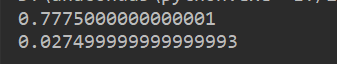这里正确标签用one-hot编码，y用softmax输出表示。第一个例子的正确标签为2，对应的概率为0.05，第二个例子对应标签为0.8.可以发现第二个例子的损失函数的值更小，和训练数据更吻合。
2.交叉熵误差（cross entropy error）
除了均方误差之外，交叉熵误差也常被用做损失函数。表达式为：

这里，log表示以e为底的自然对数（)。是神经网络的输出，是训练数据的标签值。并且，中只有正确解标签的索引为1，其他均为0（one-hot）表示。因此这个式子实际上只计算对应正确解标签的输出的自然对数。
自然对数的图像为：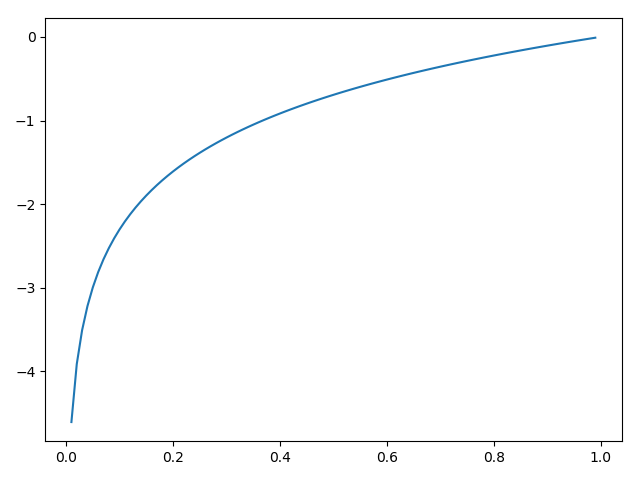所以输出的概率越大对应损失函数的值越低。
代码实现交叉熵误差：
def cross_entropy_error(y, t):
delta = 1e-7
return -np.sum(t * np.log(y + delta))
这里设置delta，是因为当出现log(0)时，np.log(0)会变为负无穷大。所以添加一个微小值可以防止负无穷大的发生。
还用刚刚那个例子：
t = [0, 1, 0, 0]
y = [0.1, 0.05, 0.05, 0.8]
print(cross_entropy_error(np.array(y), np.array(t)))

t = [0, 1, 0, 0]
y = [0.1, 0.8, 0.05, 0.05]
print(cross_entropy_error(np.array(y), np.array(t)))
输出为：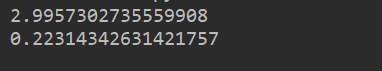可以看出输出值的概率越大损失值就越小。

交叉熵误差的改进：
前面介绍了损失函数的实现都是针对单个数据。如果要求所有训练数据的损失函数的总和，以交叉熵为例，可以写成下面的式子：

这里，假设数据有N个，表示第n个数据的第k个元素的值。式子虽然看起来复杂，其实只是把求单个数据的损失函数扩大到了N份数据，不过最后要除以N进行正规化。
通过除以N，可以求单个数据的“平均损失函数”。通过这样的平均化，可以获得和训练数据的数量无关的统一指标。比如，即使训练数据有100或1000个，也可以求得单个数据的平均损失函数。
所以对之前计算单个数据交叉熵进行改进,可以同时处理单个数据和批量数据:
def cross_entropy_error(y, t):
if y.ndim == 1:
t = t.reshape(1, t.size)
y = y.reshape(1, y.size)

batch_size = y.shape
delta = 1e-7
return -np.sum(t * np.log(y + delta)) / batch_size

但是，对于训练数据不是one-hot表示，而是普通标签表示怎么办呢（例如一批处理5个数据的标签值为[2,5,7,3,4]）。输出的数组是5行N列的，这里以手写数字识别为例所以N=10。所以我们计算的交叉熵误差其实计算的是对应每一行，其中某一列的对数之和。例如标签值[2,5,7,3,4]，选择的是输出结果的第一行第2个，第二行第5个，第三行第7个...可能表达的不是很清楚，看下代码实现应该好多了。
def cross_entropy_error(y, t):
if y.ndim == 1:
t = t.reshape(1, t.size)
y = y.reshape(1, y.size)

batch_size = y.shape
delta = 1e-7
return -np.sum(np.log(y[np.arange(batch_size), t] + delta)) / batch_size
也就是说，这里的标签值是作为输出数组的索引，用于定位。


展开全文均方误差 python 损失函数
• 4.2.4　mini-batch 版交叉熵误差的实现 one-hot格式的监督数据 def cross_entropy_error(y, t): if y.ndim == 1: #一维数组要把第一维放到第二维，表示只有一条数据 t =...

https://blog.csdn.net/weixin_43114885/article/details/90378914
4.2.4　mini-batch 版交叉熵误差的实现

one-hot格式的监督数据
def cross_entropy_error(y, t):     if y.ndim == 1: #一维数组要把第一维放到第二维，表示只有一条数据         t = t.reshape(1, t.size) #reshape函数代两维的参数         y = y.reshape(1, y.size)
batch_size = y.shape #记下第一维的数值，表示有多少条数据     return -np.sum(t * np.log(y + 1e-7)) / batch_size
t为（batch_size,10), y 为（batch_size,10)
t*logy   结果还是（batch_size,10)
np.sum 后成为一个数值
y=np.array([[0,1,0.1,0,0,0,0,0,0,0],             [0,0,0.2,0.8,0,0,0,0,0,0]]) t=np.array([[0,1,0,0,0,0,0,0,0,0],             [0,0,0,1,0,0,0,0,0,0]])#one-hot

batch_size =y.shape  print( batch_size ) #batch_size =2 r= -np.sum(t * np.log(y + 1e-7)) / batch_size  print(r) #0.11157166315711126

非one-hot格式的监督数据
def cross_entropy_error(y, t):     if y.ndim == 1:         t = t.reshape(1, t.size)         y = y.reshape(1, y.size)
batch_size = y.shape     return -np.sum(np.log(y[np.arange(batch_size), t] + 1e-7))/ batch_size #
import numpy as np
y=np.array([[0,1,0.1,0,0,0,0,0,0,0],                         [0,0,0.2,0.8,0,0,0,0,0,0]]) t_onehot=np.array([[0,1,0,0,0,0,0,0,0,0],                                [0,0,0,1,0,0,0,0,0,0]])#one-hot t = t_onehot.argmax(axis=1)#非one-hot print(t)#[1 3] batch_size = y.shape print(batch_size)#2 k=y[np.arange(batch_size), t] # [y[0,1] y[1,3]] print(k)#[1.  0.8] r=-np.sum(np.log(y[np.arange(batch_size), t] + 1e-7))/ batch_size print(r)#0.11157166315711126

t为（batch_size,1), y 为（batch_size,10)y[np.arange(batch_size), t]   结果是一维的（有batch_size个值） np.sum 后成为一个数值

import numpy as np a=np.array([1,2,3,4]) print(a) # [1 2 3 4] b=a.reshape(1,a.size) print(b)#[[1 2 3 4]]
展开全文• 神经网络NN以某个指标为线索...很多函数都可以作为损失函数loss function，但常用的是均方误差和交叉熵误差等。 一、均方误差Mean Squared Error E=12∑k(yk−tk)2E=\frac12\sum_{k}(y_k-t_k)^2E=21​k∑​(yk​−t...损失函数
• 交叉熵误差（cross entropy error）： log表示ln yk：神经网络输出 tk：正确解的标签 tk一般采用one-hot表示，这样乘积的结果由正确解对应的值所决定 yk越小，E越大 cross_entropy_error.py # oding:utf-8 ...python
• 参照书写的一个简单的SGD正向传播2层神经网络，读入mnist数据格式为1002828，激活函数为sigmoid，中间层输入展开为784(28*28)，输出层为10(对应0-9数字)，但最后的结果学习无法进行，交叉熵误差一直波动没法稳定下降...深度学习
• 神经网络的学习中的“学习”是指从训练数据中自动获取最优权重参数的过程。 为了使神经网络能进行学习，将导入...这个损失函数可以使用任意函数，但一般用均方误差和交叉熵误差等。 损失函数是表示神经网络性能的“恶
• #交叉熵误差 #设“2”为正确解 t = [0, 0, 1, 0, 0, 0, 0, 0, 0, 0] #定义mse函数 def cross_entropy_error(y, t): delta = 1e- 7 return -np.sum(t * np.log(y + delta)) #例1：”2“的概率最高的情况(0.6) y =...python 深度学习
• DL之SoftmaxWithLoss：SoftmaxWithLoss算法(Softmax函数+交叉熵误差)简介、使用方法、应用案例之详细攻略 目录 SoftmaxWithLoss算法简介 1、Softmax-with-Loss层的计算图 2、正向传播 3、反向传播 4...
• 笔记(八)，其中 ...Softmax-with-Loss 层（Softmax 函数和交叉熵误差）的计算图如下： 其中Softmax 函数（笔记四） 可以减去输入的最大值来防止溢出 a = np.array([1010, 1000, 990]) y=np.ex...
• 均方误差（Mean Squared Error，MSE）。 流行于8、90年代，其设计如下： MSE(ŷ&amp;amp;amp;amp;amp;amp;amp;amp;amp;nbsp;)=1n∑k=1n(ŷ&amp;amp;amp;amp;amp;amp;amp;amp;amp;nbsp;−y)2MSE(y^)=1n......